## 3.2.1 Perturbations to a Random Variable

First we discuss a situation in which the mean of a random variable is obtained in an uncomplicated setting. Then we add one or more complications that result in the addition of perturbation terms to the original random variable. The question of interest is: How do we find the mean of the random variable with the added perturbation term(s)?

Example 6: Barriers to Travel

The method is illustrated by an example involving barriers to travel. Suppose that we reanalyze the rectangular response district (with a randomly placed response unit) described in Exercise 3.1. We wish to discover how a barrier that impedes travel increases the mean travel time. For convenience, we deal directly with travel distance, which is assumed to be right-angle.

Solution

We again assume that the incident's position (X1, Y1) and response unit's position (X2, Y2) are uniformly independently distributed over the district. (In Exercise 3.1, the incident was a medical emergency and the response unit was an ambulette.) We know now that

E[D] = 1/3(X0 + Y0)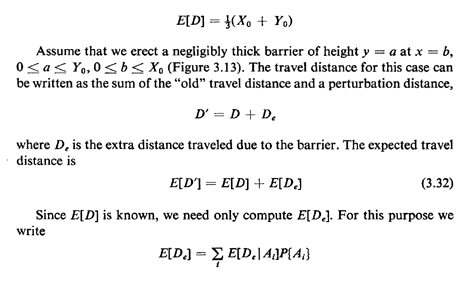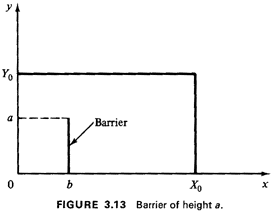where the events Ai are mutually exclusive and collectively exhaustive. In particular, consider the eventsThus, using (3.12) and (3.32)-(3.34), we have the desired result: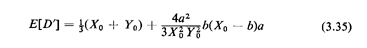The second term on the right-hand side is the perturbation term.

To gain insight into the magnitude of the perturbation term, suppose that we have a unit-area square district with a barrier originating at a boundary halfway point,This function is plotted for 0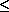a1 in Figure 3.14. Note that a barrier of height a = 1/2, say, yields a mean travel distance of 0.708, which is only about 6.3 percent greater than the mean travel distance with no barrier (0.667).

We could use the same method to find the average travel distance for the case of a barrier extending completely from y = 0 to y = Y0 with one crossing point at y = a (e.g., a river at x = b with a bridge at y = a). The simplest way to do this is to add sequentially a second perturbation distance so that

D'' = D' + D'e

where D'e. is the extra distance traveled compared to the distance D'.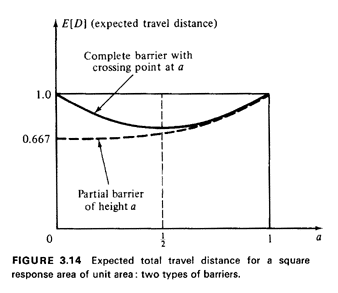Exercise 3.2: River with One Bridge Using symmetry, show that for this case (the complete barrier with one crossingpoint)This function is also plotted in Figure 3.14.

The variance of D' in our original example is more difficult to obtain due to "cross terms" between D and De. Substituting directly into the definition of variance, we find that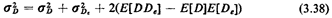The variance of De is directly obtainable using the argument above (solving first for E[D2e] by conditioning on the event De > 0). However, the term in parentheses in general is not equal to zero, because of the dependence between D and D,. This dependence can be seen intuitively by considering the conditional information De = 2a: then both customer and server are located on opposite sides of the barrier at y = 0 and D is (conditionally) distributed as {(|X1 - X2|) | Min [X1, X2]b, Max [X1,X2] > b} rather than |X1 - X2 + |Y1 - Y2 (unconditioned). Thus, while derivation of the variance presents no conceptual problems, we will limit our work with perturbations to a random variable to obtaining the mean of the new random variable.

We may summarize (and generalize) the approach as follows:

1. Express the random variable of interest W' as the sum of an easy-toanalyze (simple) random variable W and one or more perturbation random variables W'e, W''e....(The perturbation random variables will usually have a positive probability of assuming the value zero.)
2. Compute the mean of W' by summing the mean of the simple random variable and the conditional means of each perturbation random variable, given that it is positive, multiplied by the probability that it is positive: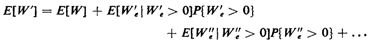Further work: Problems 3.13-3.16,

4 Points (x=b,y<a) are grouped arbitrarily with all the points to the left of the barrier.